Area of segment of circle and length of arc

Chapter 12 Class 10 Areas related to Circles
Concept wise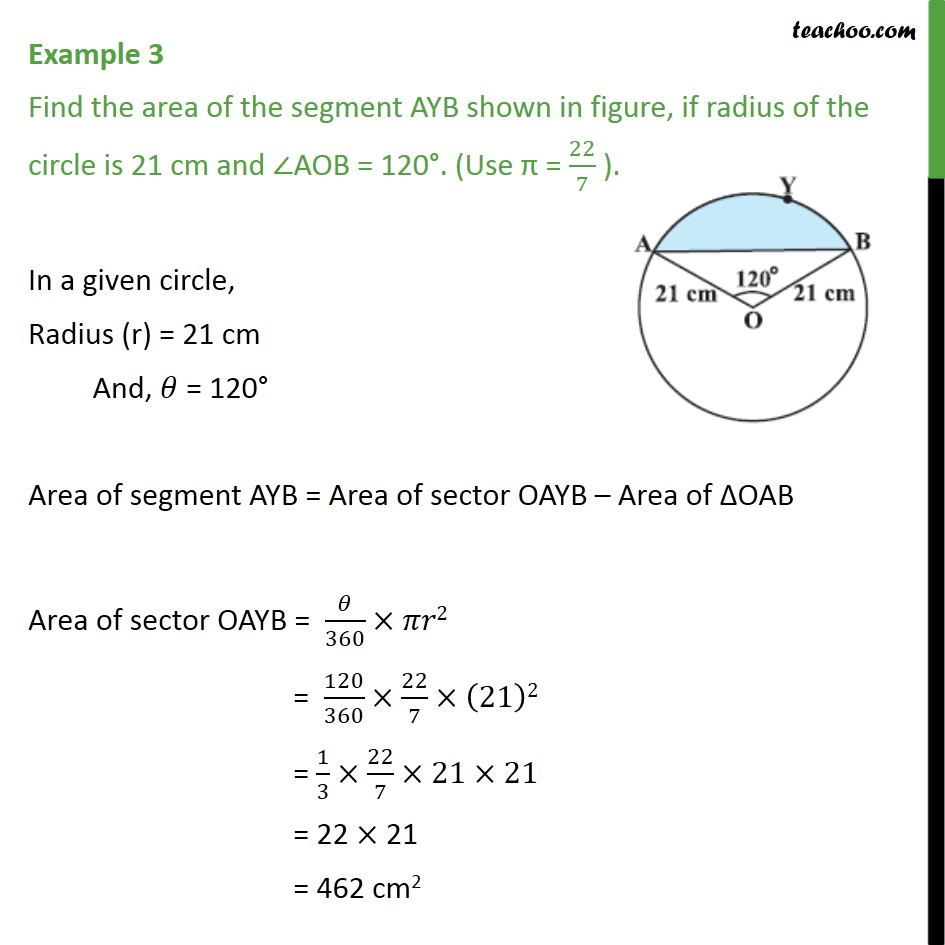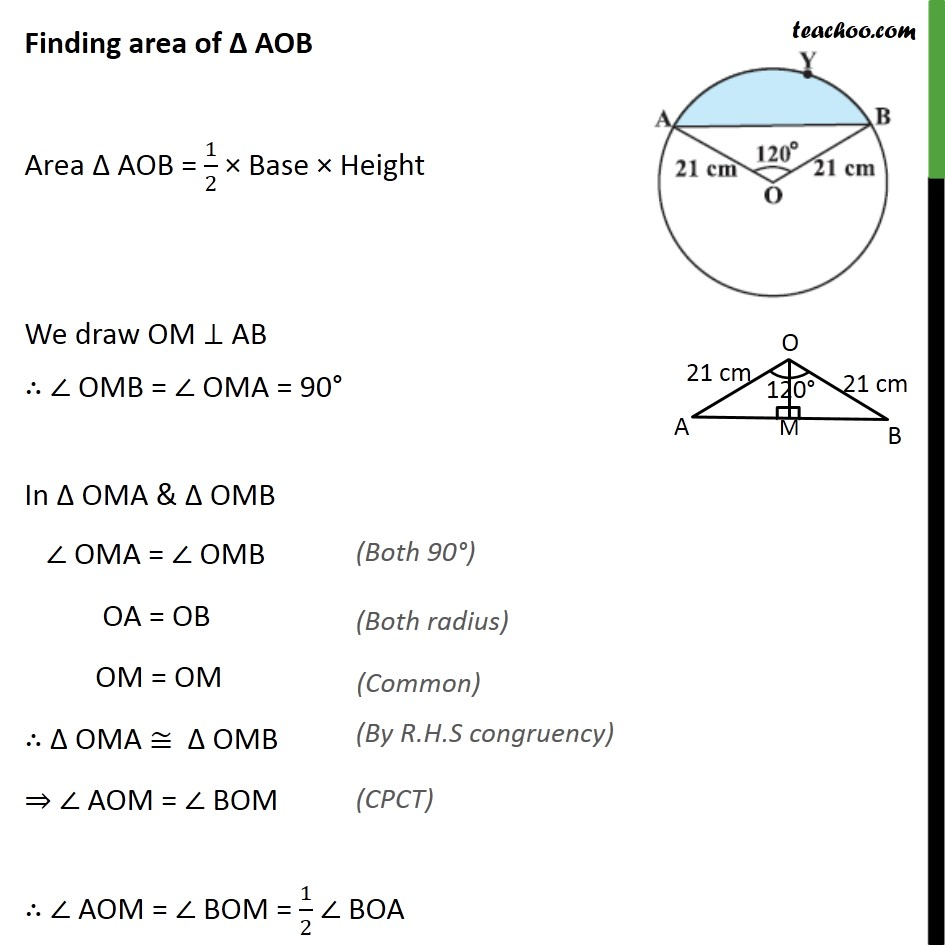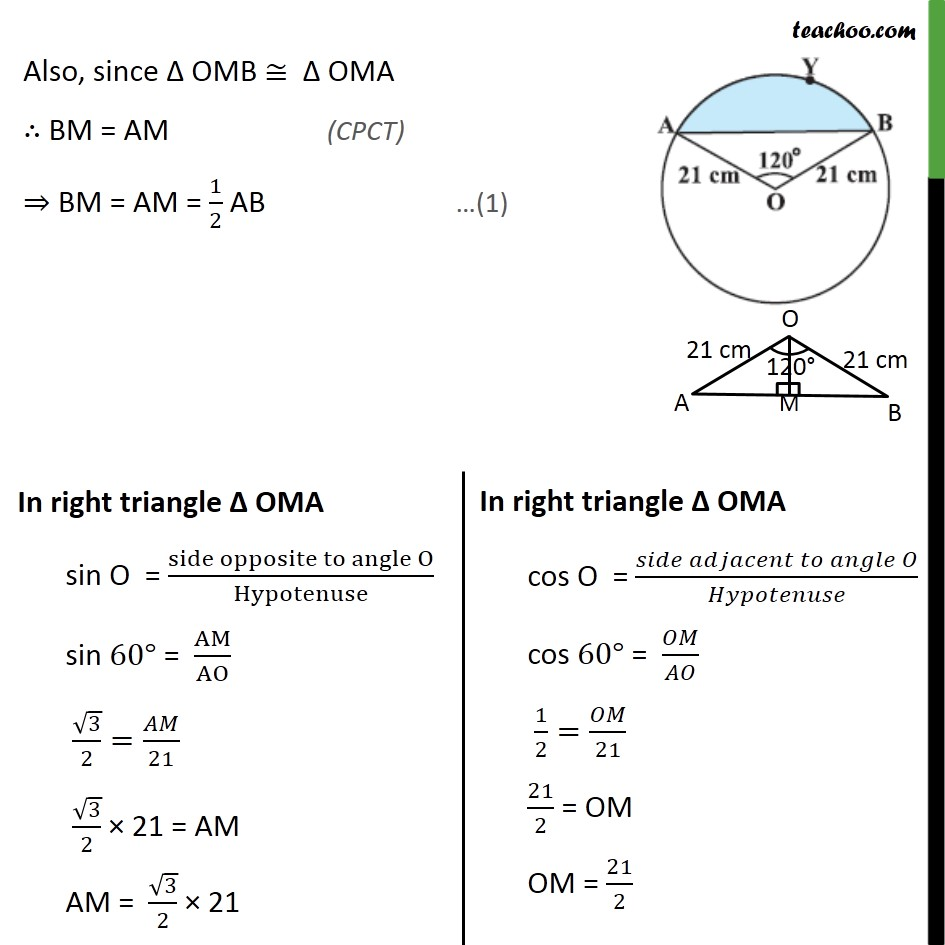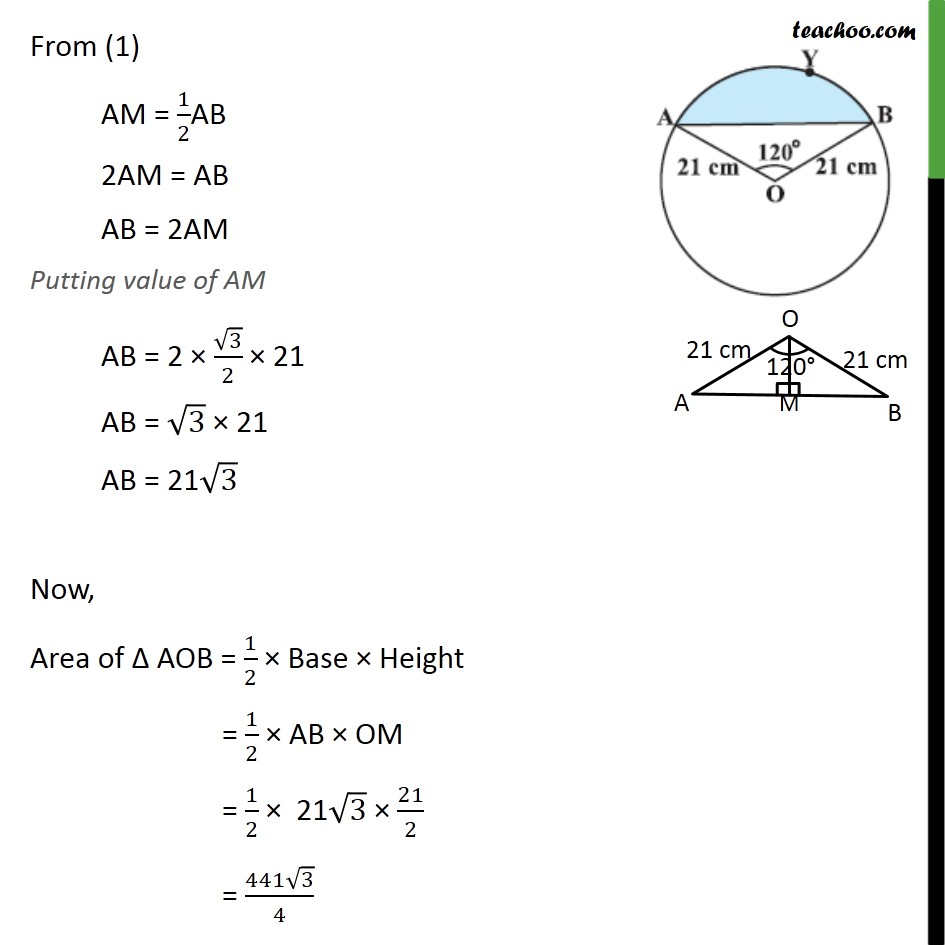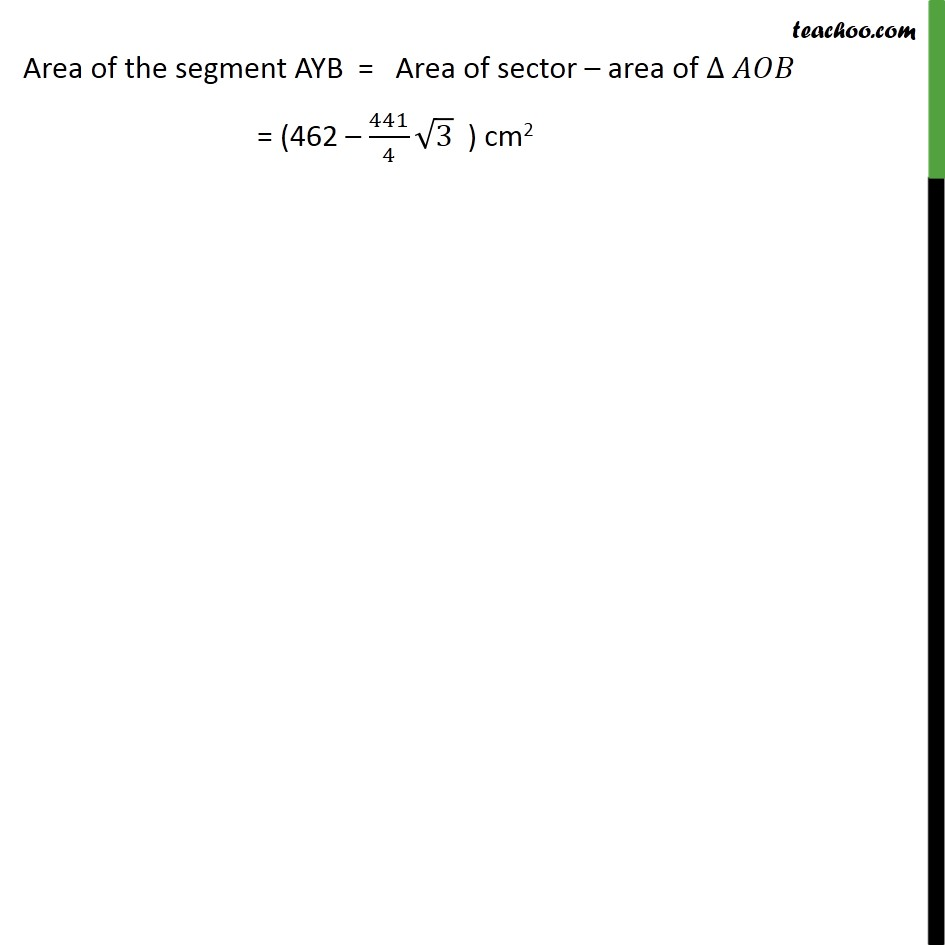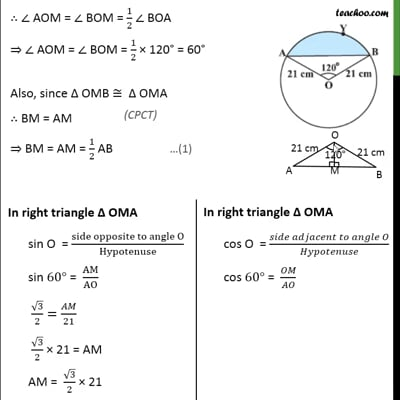This video is only available for Teachoo black users

Introducing your new favourite teacher - Teachoo Black, at only ₹83 per month

### Transcript

Example 3 Find the area of the segment AYB shown in figure, if radius of the circle is 21 cm and ∠AOB = 120°. (Use π = 22/7 ). In a given circle, Radius (r) = 21 cm And, 𝜃 = 120° Area of segment AYB = Area of sector OAYB – Area of ΔOAB Area of sector OAYB = 𝜃/360×𝜋𝑟2 = 120/360×22/7×(21)2 = 1/3×22/7×21×21 = 22 × 21 = 462 cm2 Finding area of Δ AOB Area Δ AOB = 1/2 × Base × Height We draw OM ⊥ AB ∴ ∠ OMB = ∠ OMA = 90° In Δ OMA & Δ OMB ∠ OMA = ∠ OMB OA = OB OM = OM ∴ Δ OMA ≅ Δ OMB ⇒ ∠ AOM = ∠ BOM ∴ ∠ AOM = ∠ BOM = 1/2 ∠ BOA Also, since Δ OMB ≅ Δ OMA ∴ BM = AM ⇒ BM = AM = 1/2 AB From (1) AM = 1/2AB 2AM = AB AB = 2AM Putting value of AM AB = 2 × √3/2 × 21 AB = √3 × 21 AB = 21√3 Now, Area of Δ AOB = 1/2 × Base × Height = 1/2 × AB × OM = 1/2 × 21√3 × 21/2 = (441√3)/4 Area of the segment AYB = Area of sector – area of ∆ 𝐴𝑂𝐵 = (462 – 441/4 √3 ) cm2